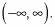##### Linear Algebra For DummiesYou can transform the graph for tangent and cotangent vertically, change the period, shift the graph horizontally, or shift it vertically. However, you should take each transformation one step at a time.

For example, to graph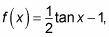1. Sketch the parent graph for tangent.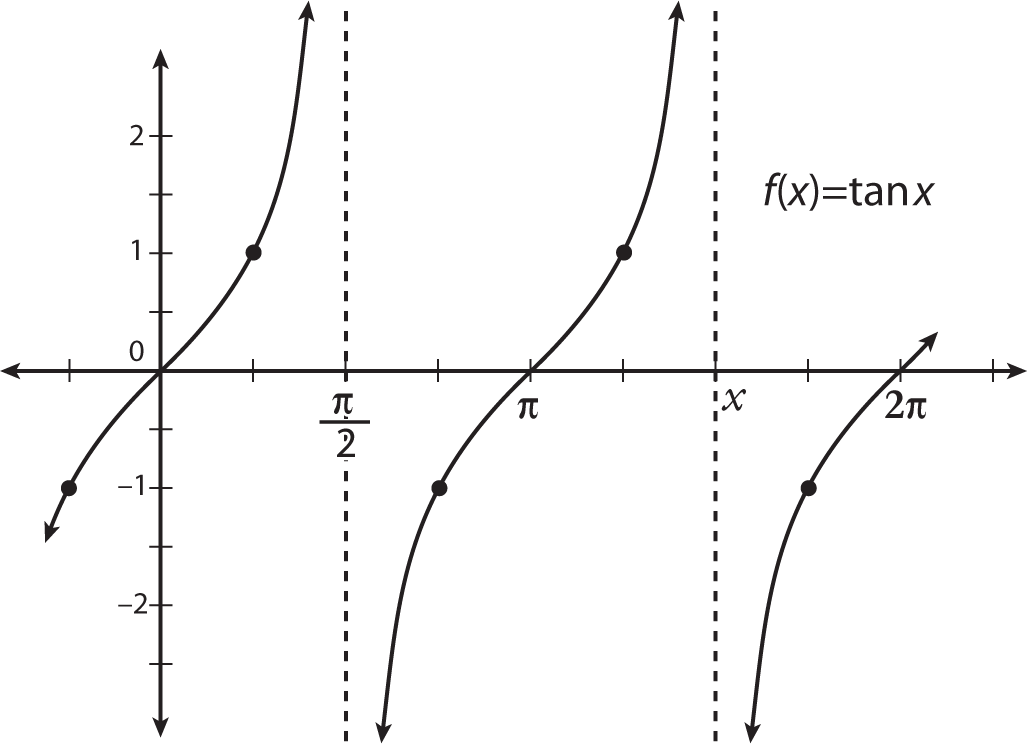2. Shrink or stretch the parent graph.

The vertical shrink is 1/2 for every point on this function, so each point on the tangent parent graph is half as tall.

Seeing vertical changes for tangent and cotangent graphs is harder, but they're there. Concentrate on the fact that the parent graph has points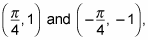which in the transformed function become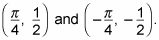As you can see in the figure, the graph really is half as tall!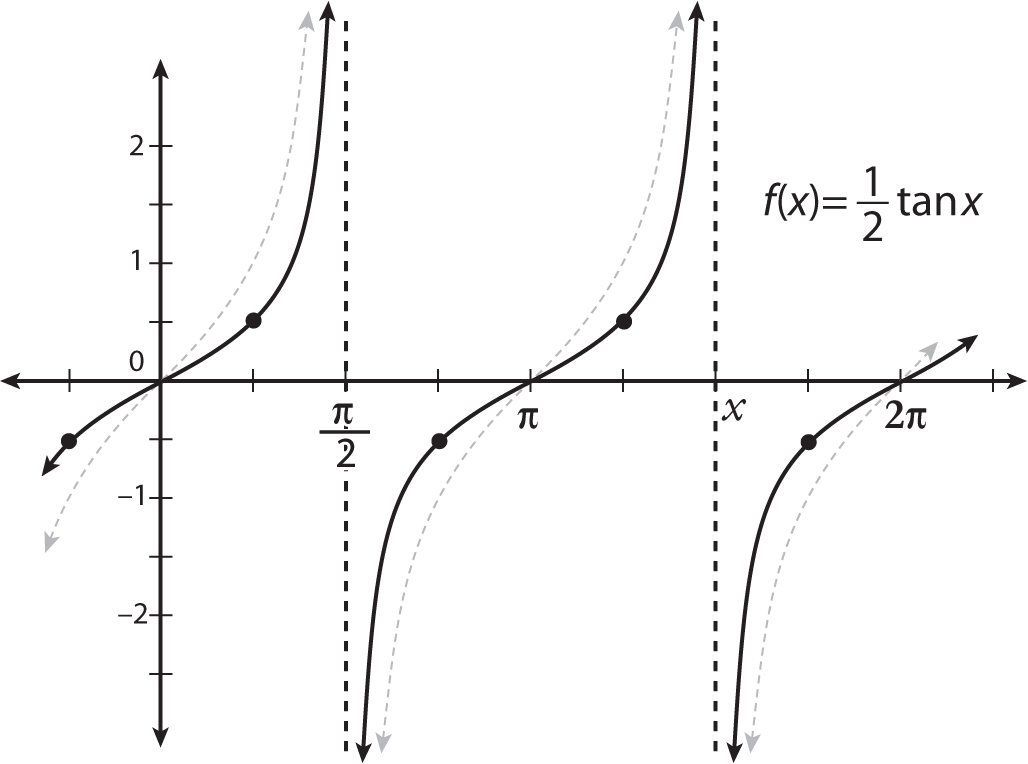The graph of y = (1/2)tanx.
3. Change the period.

The constant 1/2 doesn't affect the period. Why? Because it sits in front of the tangent function, which only affects vertical, not horizontal, movement.

4. Shift the graph horizontally and vertically.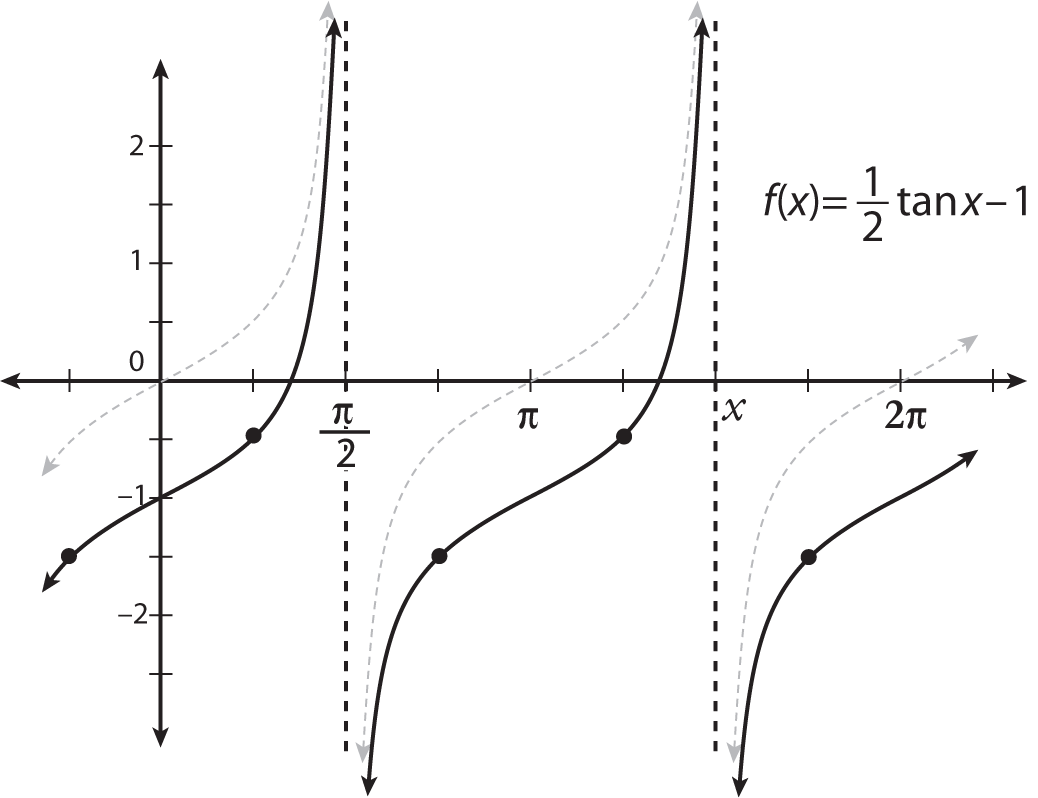This graph doesn't shift horizontally, because no constant is added inside the grouping symbols (parentheses) of the function. So you don't need to do anything horizontally. The – 1 at the end of the function is a vertical shift that moves the graph down one position. The figure shows the transformed graph of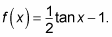5. State the transformed function's domain and range, if asked.

Because the range of the tangent function is all real numbers, transforming its graph doesn't affect the range, only the domain. The domain of the tangent function isn't all real numbers because of the asymptotes. The domain of the example function hasn't been affected by the transformations, however. Where n is an integer,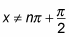Now that you've graphed the basics, you can graph a function that has a period change, as in the function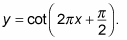You see a lot of pi in that one. Relax! You know this graph has a period change because you see a number inside the parentheses that's multiplied by the variable. This constant changes the period of the function, which in turn changes the distance between the asymptotes. In order for the graph to show this change correctly, you must factor this constant out of the parentheses. Take the transformation one step at a time:

1. Sketch the parent graph for cotangent.

2. Shrink or stretch the parent graph.

No constant is multiplying the outside of the function; therefore, you can apply no shrink or stretch.

3. Find the period change.

You factor out the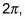which affects the period. The function now reads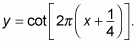The period of the parent function cotangent is pi. Therefore, you must divide pi by the period coefficient, in this case 2pi. This step gives you the period for the transformed cotangent function: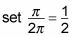so you get a period of 1/2 for the transformed function. The graph of this function starts to repeat at 1/2, which is different from pi/2, so be careful when you're labeling your graph.

This period isn't a fraction of pi; it's just a rational number. When you get a rational number, you must graph it as such. The figure shows this step.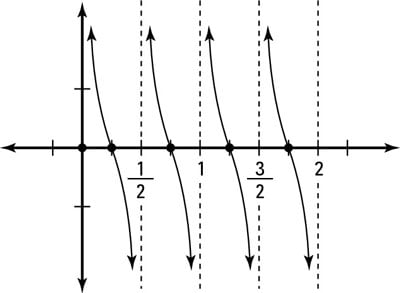Graphing of y(x) = cot 2pi x shows a period of 1/2.
4. Determine the horizontal and vertical shifts.

Because you've already factored the period constant, you can see that the horizontal shift is to the left 1/4. The next figure shows this transformation on the graph.

No constant is being added to or subtracted from this function on the outside, so the graph doesn't experience a vertical shift.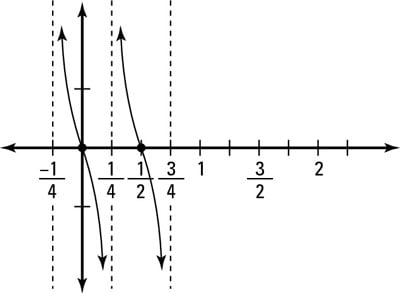The transformed graph of y(x) = cot 2pi(x + 1/4).
5. State the transformed function's domain and range, if asked.

The horizontal shift affects the domain of this graph. To find the first asymptote, set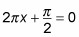(setting the period shift equal to the original first asymptote). You find that x = –1/4 is your new asymptote. The graph repeats every 1/2 radians because of its period. So the domain is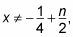where n is an integer. The graph's range isn't affected: